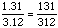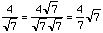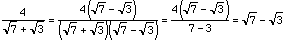Date: Mon, 25 Jan 1999 16:10:26 -0600 (CST) Subject: Simplifying Radicals Name: Mary Who is asking: Student Level: Secondary Question: I would like to know how to simplify this question: ``` 4 __________________________ squareroot7 + squareroot3 ``` I know the answer is (sqrt7 - sqrt3) but i would really love to know how to get that answer!! Thanks. Mary Hi Mary Some people would argue that the expression is already simplified but in order to get the required answer you need to perform a process called "rationalizing the denominator". This is similar to the process that is used to "simplify" a fraction when the denominator is a decimal. For exampleHere both the numerator and denominator have been multiplied by 100 to turn the denominator into a natural number. Likewise to simplify 4/sqrt7 you multiply the numerator and denominator by sqrt7 to getThe simplest way to turn a binomial denominator with radical(s) into a rational number is to multiply the binomial by the same binomial expression with the opposite sign (+ instead of - or vice versa), called its conjugate. Here you will mult the numerator and denominator by (sqrt7 - sqrt3). SoCheers Jack and Penny Go to Math Central To return to the previous page use your browser's back button.